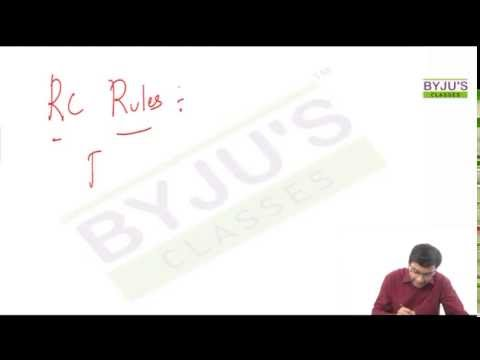# Reading Comprehensions Rules for GMAT exam

Let’s discuss a Mathematical approach to answer reading comprehensions in GMAT! You won’t get much time during the examination to read all the paragraph and answer the questions. Hardly few seconds will be available for you to answer the questions. So how do we do it? Here we go with easy reading comprehensions rules for GMAT Exam.

Usual approach to solve a problem in mathematics as follows:
1. Understand the question
2. Apply formula and get the answer
We are going to use this approach in Reading comprehension.The challenge in Reading comprehension are
1. Understanding the passage
2. Formulate the answer that is tricky